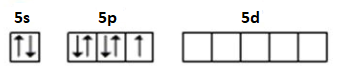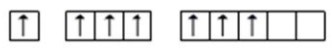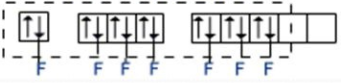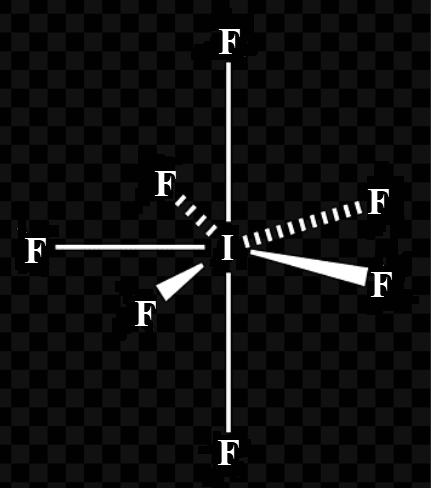QuestionAnswers

# For the molecule of $I{{F}_{7}}$.(a)State the hybridisation of the central atom.(b)State the geometry of the molecule.

Hint: Hybridization of a molecule is determined using the postulates of the Valence band theory and its geometry can be formulated by using the VSEPR rule. The geometry depends on the no. of lone pairs and bond pairs.

(a)Hybridization is when the concept of atomic orbitals fuse to make new hybridized orbitals, which successively influences molecular geometry and bonding properties. Hybridization is also an expansion of the valence bond theory.
According to Valence band theory the metal atom or ion in the influence of ligands uses its (n-1)d, ns, np, or ns, np, nd orbitals for hybridization to yield a group of equivalent orbitals of definite geometry like octahedral, tetrahedral, square planar then on.(Here ligands refers to ion or molecule, which donates a pair of electron to the central metal These hybrid orbitals are allowed to overlap with ligand orbitals which will donate electron pairs for bonding.
Hybridisation = Number of valence electrons on central atom + Number of monovalent surrounding atoms (like hydrogen and halogen)- positive charge (if on complex) + anionic charge (if on complex) and divide this whole by 2.
Now, for $I{{F}_{7}}$: Hybridisation = 7 (number of valence electrons on I-atom) + 7 (number of surrounding halogen atoms) -0+0 and divide this whole by 2. The element Iodine has 7 lone electrons. When it is bonded to seven Fluorine atoms through sigma bonds, the total number of electron pairs around Iodine becomes 7 with no lone pair of el
(Ground State)(Excited State)$s{{p}^{3}}{{d}^{3}}$- Hybridization ($I{{F}_{7}}$)(Ground State)
(Excited State)
$s{{p}^{3}}{{d}^{3}}$- Hybridization ($I{{F}_{7}}$)

Thus, the hybridisation of $I{{F}_{7}}$is $s{{p}^{3}}{{d}^{3}}$ with no lone pair of electrons and this corresponds to pentagonal bipyramidal geometry.(b) Iodine heptafluoride, $I{{F}_{7}}$, may be an example of a pentagonal bipyramidal geometry. VSEPR rules suggest seven electron pairs. These are made up from six bonding pairs and one lone pair.

Note:
-VSEPR theory has certain postulates which one must follow to deduce the geometrical structure of a compound.
-It can be noted that sigma bonds involve the head-to-head overlapping of atomic orbitals whereas pi bonds involve parallel overlapping.